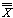# Statistics

The Statistics window gives the operator a current reading of relevant numerical information.

## Statistics Window Items

The Statistics window gives the operator a current reading of relevant numerical information.
The following items will be found in the Statistics window:

• Subgroup number (k).
• Subgroup size (n).
• ObsCnt = Observation Count = number of individual observations made.
• XDBar == X -double bar = the mean of the subgroup averages.
• Rbar = average of the subgroup ranges.
• s (all) = s
 s == current standard deviation of inspection run
• RBar/d2 = estimated standard deviation
• Min = minimum observation value
• Max = maximum observation value
• Mean +/- 3,4,6s = control limits for XBar chart
• Defect Ratio = percentage of measurements outside of tolerance
• PPM = parts per million outside of tolerance
• Cp = potential process capability index = (UTL-LTL)/6s
where s = stnd.dev = RBar/d2
• Cpk = potential process performance index = Zmin/3 where Zmin = min {(UTL –)/s, (– LTL)/s}, where s = RBar/d2
• CPL = (– LTL)/3s, where s = RBar/d2
• CPU = (UTL –)/3s, where s = RBar/d2• Ppk = actual process performance index = Zmin/3 where Zmin = min {(UTL –)/s, (– LTL)/s}, where